# Gauss’ Goal Generation

Using gaussian process models for hospital goal setting
bayes
stan
healthcare
rstats
Author

Mark Rieke

Published

March 19, 2023

Code
library(tidyverse)
library(riekelib)

This past week, I’ve been living and breathing (and dying, a little bit) with goals. Memorial Hermann’s mission is to provide safe, personalized, efficient, and caring healthcare and part of this endeavor is striving to improve each patient’s experience (look ma, I paid attention during corporate culture day). As such, we set goals for improving metrics measuring patient satisfaction. One of my responsibilities as a consumer experience (CX) analyst is to model our net promoter score (NPS) so that the goals we suggest are ambitious yet achievable. In preparation for the new fiscal year in July, I’ve been making updates to the goal model. I recently reached a mid-model stopping point, so now is a good time to take a mental break, recollect my thoughts, and give a peek at what I’ve got cooking under the hood of the model.

## Foundational features: the current model

The model for this current year is serviceable, but fairly basic. For each unit, we estimate a distribution of possible outcomes based on the campus, service area (Inpatient, Outpatient, Emergency, etc.), and previous year’s score. From this distribution, we’re able to set three different goal levels (threshold, target, and distinguished), which can then be rolled up into summary scores at various levels (campus & service area, system & service area, system overall, etc.). This certainly works and provides a more data-informed methodology for goal setting than simply asserting that every location needs to improve by X% to meet goals (areas with small sample sizes can trivially crush or be crushed by goals due to expected sampling variation!), but there are ways we can improve.

## Prototyping predictions: expanding the model

The (in progress) updated model builds on the foundation set by the last model — we’re still estimating a distribution of possible scores at three levels, then rolling up to summary levels. The big addition to the new model is a time series component measuring NPS at each quarter, which is implemented via a gaussian process. There are other ways to model a time series, but I’ve elected to use a gaussian process for a couple reasons:

• Gaussian processes allow for scores to be correlated over time. This means that scores in each quarter are likelier to be similar to the previous quarter’s score rather than a score from five quarters ago.
• Gaussian processes are stable over time (at least with the kernel I used). This is something I’ve seen in the context of hospital patient satisfaction scores (in the very least, the changes over time fall within an uncertainty interval roughly indistinguishable from stable).
• Gaussian processes allow us to apply prior information to events in the future that we don’t yet have data for (see Drew Linzer’s 2013 paper describing his election model for an example). This is important as Memorial Hermann transitions to Epic next year, which we expect to have a slight negative impact on patient experience.
• You are what you read — I read a lot of Gelman, who is a fan of gaussian processes, so I suppose some of that has rubbed off on me.
• They just seem rad as hell.

I’ll get into all the fun, math-y details below, but it’s worth highlighting that the model I describe did not emerge fully formed. Following Gelman’s idea of a Bayeian workflow, this model was built iteratively and there were a lot (like, really, a lot) of failed models that came before this one. Furthermore, there’s still over a quarter of data to collect before the end of the fiscal year and a few un-implemented features, so this definitely isn’t the final version.

## Math for days: model specification

For each hospital’s service area, $$i$$, the number of promoter, passive, and detractor responses in a quarter, $$j$$, can be described with a multinomial:

\begin{align*} R_{ij} & \sim \text{Multinomial}(n_{ij}, p_{ij}) \end{align*}

$$p_{ij}$$ is a probability vector with individual probabilities for each of the possible categories the patients can select:

\begin{align*} p_{ij} & = \langle p_{\text{promoter}},\ p_{\text{passive}},\ p_{\text{detractor}} \rangle_{ij} \end{align*}

The categories are ordered (promoter > passive > detractor), so we can link the categorical probabilities to a single linear model, $$\phi$$, via the cumulative probabilities, $$q$$, and $$k$$ cutpoints, $$\kappa$$. I’ve described this in a bit more detail in a previous post, so I’ll just leave you with the math, here. Just note that as $$\phi$$ increases, so too does the expected NPS.

\begin{align*} p_{\text{detractor},\ ij} & = q_{1,\ ij} \\ p_{\text{passive},\ ij} & = q_{2,\ ij} - q_{1,\ ij} \\ p_{\text{promoter},\ ij} & = 1 - q_{2,\ ij} \\ \text{logit}(q_{kij}) & = \kappa_k - \phi_{ij} \\ \phi_{ij} & = \text{some linear model} \end{align*}

This is where things get interesting! Let’s add some terms to the linear model. I include hierarchical terms for the campus and service area as well as an interaction between the two (the interaction allows for additional variation beyond what the campus and service terms alone would allow). These terms don’t change over time and deviations from the set value over time are handled via a gaussian process parameter, $$\beta_{ij}$$.

\begin{align*} \phi_{ij} & = \alpha + z_{\text{campus}[i]}\ \sigma_{\text{campus}} + z_{\text{service}[i]}\ \sigma_{\text{service}} + z_{\text{interact}[i]}\ \sigma_{\text{interact}} + \beta_{ij} \end{align*}

$$\beta_{ij}$$ could be specified using a multivariate normal distribution, but gaussian processes are a bit finnicky, so we’ll use a non-centered parameterization.

\begin{align*} \beta_{ij} & = \eta_{ij}\ L \end{align*}

Here, $$\eta_{ij}$$ is an offset parameter for each hospital’s service area $$i$$ at each quarter $$j$$ and $$L$$ is the Cholesky decomposition of the covariance matrix, which defines how similar scores are over time.

\begin{align*} L & = \text{cholesky decompose}(\Sigma) \end{align*}

The covariance between each pair of quarters is defined using an exponentiated quadratic kernel, which is based on two tuning parameters, $$\sigma_{\text{amplitude}}^2$$ and $$\sigma_{\text{length}}^2$$, and the distance in time, $$t$$, between the quarters. $$\sigma_{\text{amplitude}}^2$$ controls the range in which $$\beta_{ij}$$ might end up falling while $$\sigma_{\text{length}}^2$$ controls the time period over which scores can covary (a lengthy timescale implies that scores are slow to change while a short timescale implies that scores can change quickly). For computational efficiency, I scale the $$t$$ to be between 0 and 1. Notably, the parameters controlling the covariance matrix do not vary by campus or service. This is intentional! This implies that we think that satisfaction tends to change at similar rates (though scores can fluctuate more quickly due to sampling variation).

\begin{align*} \Sigma_{xy} & = \sigma_{\text{amplitude}}^2 \exp \left( \frac{|t_x - t_y|^2}{2 \ \sigma_{\text{length}}^2}\right) \end{align*}

Altogether, with priors, here’s the full model in all it’s wonky mathematical glory:

\begin{align*} R_{ij} & \sim \text{Multinomial}(n_{ij}, p_{ij}) \\ p_{ij} & = \langle p_{\text{promoter}},\ p_{\text{passive}},\ p_{\text{detractor}} \rangle_{ij} \\ p_{\text{detractor},\ ij} & = q_{1,\ ij} \\ p_{\text{passive},\ ij} & = q_{2,\ ij} - q_{1,\ ij} \\ p_{\text{promoter},\ ij} & = 1 - q_{2,\ ij} \\ \text{logit}(q_{kij}) & = \kappa_k - \phi_{ij} \\ \phi_{ij} & = \alpha + z_{\text{campus}[i]}\ \sigma_{\text{campus}} + z_{\text{service}[i]}\ \sigma_{\text{service}} + z_{\text{interact}[i]}\ \sigma_{\text{interact}} + \beta_{ij} \\ \beta_{ij} & = \eta_{ij}\ L \\ L & = \text{cholesky decompose}(\Sigma) \\ \Sigma_{xy} & = \sigma_{\text{amplitude}}^2 \exp \left( \frac{|t_x - t_y|^2}{2 \ \sigma_{\text{length}}^2}\right) \\ \kappa_k & \sim \text{Normal}(-1, 0.25) \\ \alpha & \sim \text{Normal}(0, 1) \\ z_{\text{campus}} & \sim \text{Normal}(0, 1) \\ z_{\text{service}} & \sim \text{Normal}(0, 1) \\ z_{\text{interact}} & \sim \text{Normal}(0, 1) \\ \sigma_{\text{campus}} & \sim \text{Gamma}(2, 3) \\ \sigma_{\text{service}} & \sim \text{Gamma}(2, 3) \\ \sigma_{\text{interact}} & \sim \text{Half-Normal}(0, 0.5) \\ \eta & \sim \text{Normal}(0, 1) \\ \sigma_{\text{amplitude}}^2 & \sim \text{Beta}(2, 25) \\ \sigma_{\text{length}}^2 & \sim \text{Beta}(2, 10) \end{align*}

There’s a folk theorem of statistical computing, which states that when you have computing problems, you likely have problems with your model. Despite having lots of data from years of patient surveys, I ran into a lot of computational problems with default and weak priors. I use a mix of gamma, beta, and half-normal distributions as priors for scale parameters to move some of the prior mass off 0 and restrict the scale range such that unreasonable values are rejected.

This is a lot funky math, which I love! But it can be pretty opaque to read through. In the next section, I’ll simulate some fake data that will (hopefully) help provide some color to the math, then fit a model to the simulated data.

## Hypothetical hospitals: simulating fake data

I can’t share real patient data, so instead I’ll simulate responses from scratch, which will help us understand the model a bit better. First, let’s get the number of quarterly responses at five hospitals’ Inpatient, Outpatient, Emergency, and Day Surgery services over the course of two years. This isn’t estimated in the model directly (for now!), but needed to estimate NPS.

Code
# set n-size helpers to simulate from
hospitals <-
tibble(hospital = paste("Hospital", LETTERS[1:5]),
hosp_n = seq(from = 6.5, to = 4.5, length.out = 5))

services <-
tibble(service = c("Inpatient", "Outpatient", "Emergency", "Day Surgery"),
service_n = c(0, 0.5, 0.25, -0.5))

# simulate number of survey returns
set.seed(30)
responses <-
crossing(hospitals,
services,
quarter = seq.Date(from = lubridate::mdy("1/1/21"),
to = lubridate::mdy("10/1/22"),
by = "quarter")) %>%
mutate(lambda = hosp_n + service_n) %>%
select(-ends_with("n")) %>%
bind_cols(n = rpois(nrow(.), exp(.$lambda))) %>% select(-lambda) # set color palette pal <- MetBrewer::MetPalettes$Egypt[]
col_ed <- pal
col_ds <- pal
col_ip <- pal
col_op <- pal

responses <-
responses %>%
mutate(color = case_match(service,
"Emergency" ~ col_ed,
"Day Surgery" ~ col_ds,
"Inpatient" ~ col_ip,
"Outpatient" ~ col_op))

# plot!
responses %>%
ggplot(aes(x = quarter,
y = n,
color = color)) +
geom_line() +
facet_wrap(~hospital) +
scale_x_date(labels = scales::label_date("%Y"),
breaks = "years") +
scale_y_continuous(labels = scales::label_comma()) +
scale_color_identity() +
theme_rieke() +
labs(title = "Hypothetical Hospitals",
subtitle = glue::glue("Quarterly ",
"**{color_text('Day Surgery', col_ds)}**, ",
"**{color_text('Inpatient', col_ip)}**, ",
"**{color_text('Outpatient', col_op)}**, and ",
"**{color_text('Emergency', col_ed)}** ",
"responses"),
x = NULL,
y = NULL)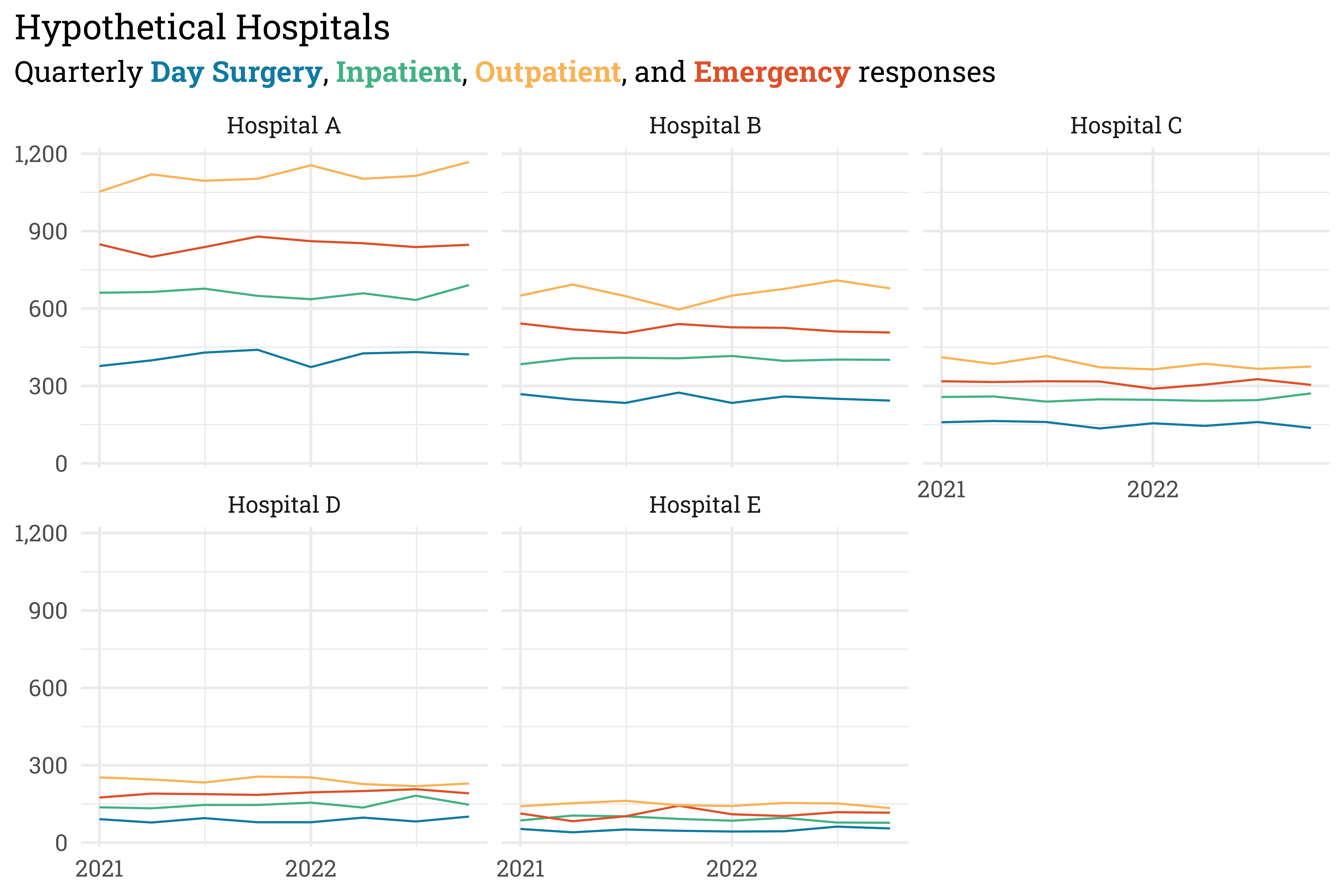Some hospitals have a far greater patient volume than others, but within each hospital, Outpatient units tend to see the most patients while Day Surgery units tend to see the fewest. Now that n-sizes are set, let’s fix some parameters so that we can simulate scores.

We’ll start by fixing the covariance matrix parameters $$\sigma_{\text{amplitude}}^2 = 0.1$$ and $$\sigma_{\text{length}}^2 = 0.1$$ (because the two years of training and one year of prediction are scaled between 0 and 1, this implies that a slow moving covariance):

Code
sigma_amplitude <- 0.1
sigma_length <- 0.1

# define a covariance function to reuse later
amplitude,
length,
delta = 1e-9) {

S <- matrix(nrow = length(x), ncol = length(x))

for (i in 1:nrow(S)) {
for (j in 1:ncol(S)) {
S[i,j] <- amplitude*exp(-(0.5/length)*(x[i] - x[j])^2)
}
S[i,i] <- S[i,i] + delta
}

return(S)

}

# time based x for this example
x <- seq(from = 0, to = 1, length.out = 12)

tibble(x = 1:12,
y = Sigma[,1]) %>%
ggplot(aes(x = x,
y = y)) +
geom_line(color = RColorBrewer::brewer.pal(4, "Dark2")) +
theme_rieke() +
labs(x = "Distance (in quarters)",
y = "Covariance") +
expand_limits(y = c(0))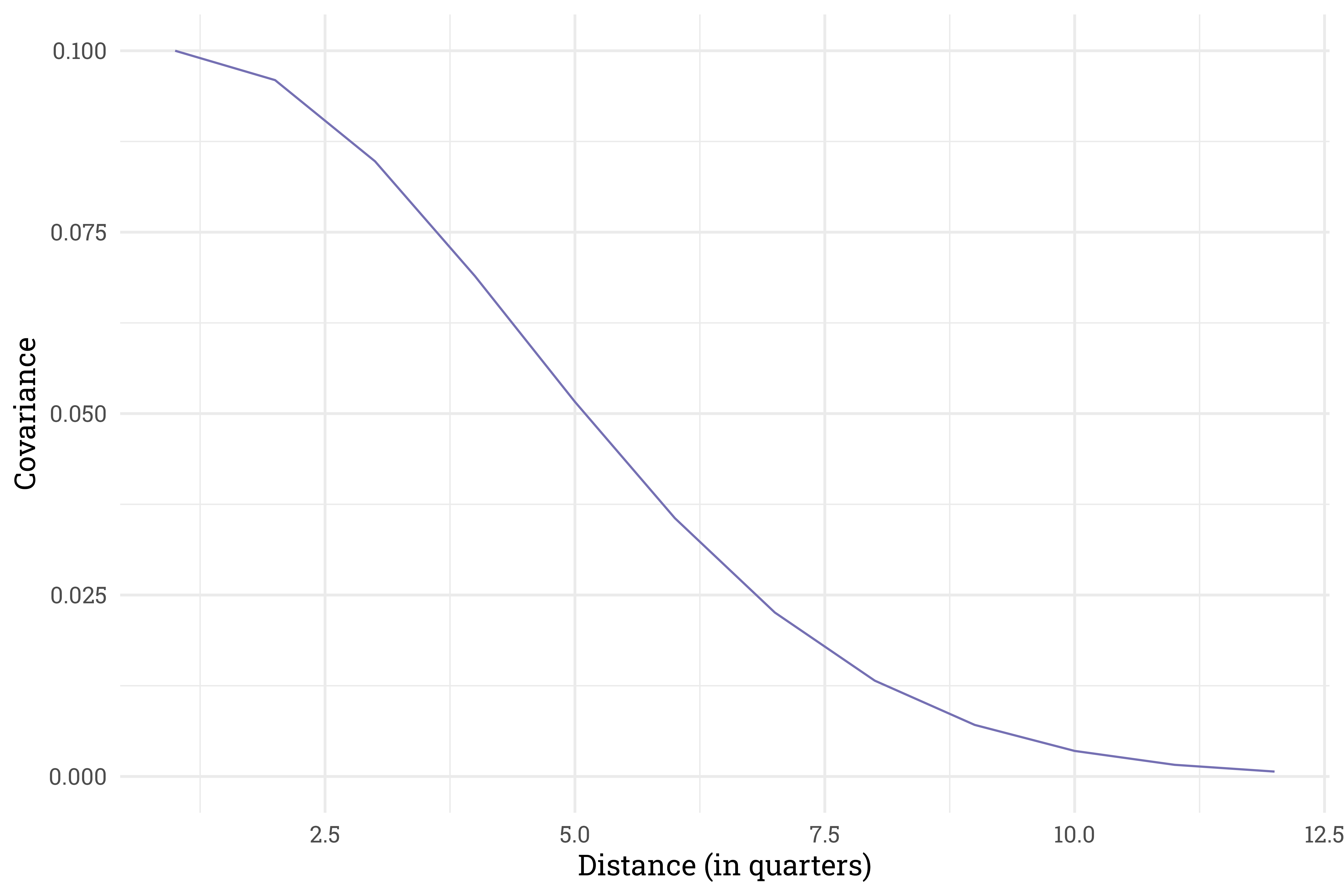Let’s see how this covariance affects a sampling of $$\beta_{ij}$$ parameters:

Code
# work through model generation process to get a list of betas
# for each quarter for each hospital/service
set.seed(31)
responses <-
responses %>%
nest(quarters = c(quarter, n)) %>%
rowwise() %>%
mutate(eta = list(rnorm(8, 0, 1))) %>%
ungroup() %>%
mutate(Sigma = list(cov_exp_quad(seq(0, 1, length.out = 12)[1:8],
sigma_amplitude,
sigma_length)),
L = map(Sigma, chol),
beta = pmap(list(L, eta), ~t(..1) %*% ..2)) %>%
select(-c(eta, Sigma, L)) %>%
mutate(beta = map(beta, ~.x[,1])) %>%
unnest(c(quarters, beta))

# plot!
responses %>%
ggplot(aes(x = quarter,
y = beta,
color = color)) +
geom_line() +
scale_color_identity() +
scale_x_date(labels = scales::label_date("%Y"),
breaks = "year") +
facet_wrap(~hospital) +
theme_rieke() +
labs(title = "Hypothetical Hospitals",
subtitle = glue::glue("Quarterly \u03B2 for ",
"**{color_text('Day Surgery', col_ds)}**, ",
"**{color_text('Inpatient', col_ip)}**, ",
"**{color_text('Outpatient', col_op)}**, and ",
"**{color_text('Emergency', col_ed)}**"),
x = NULL,
y = NULL)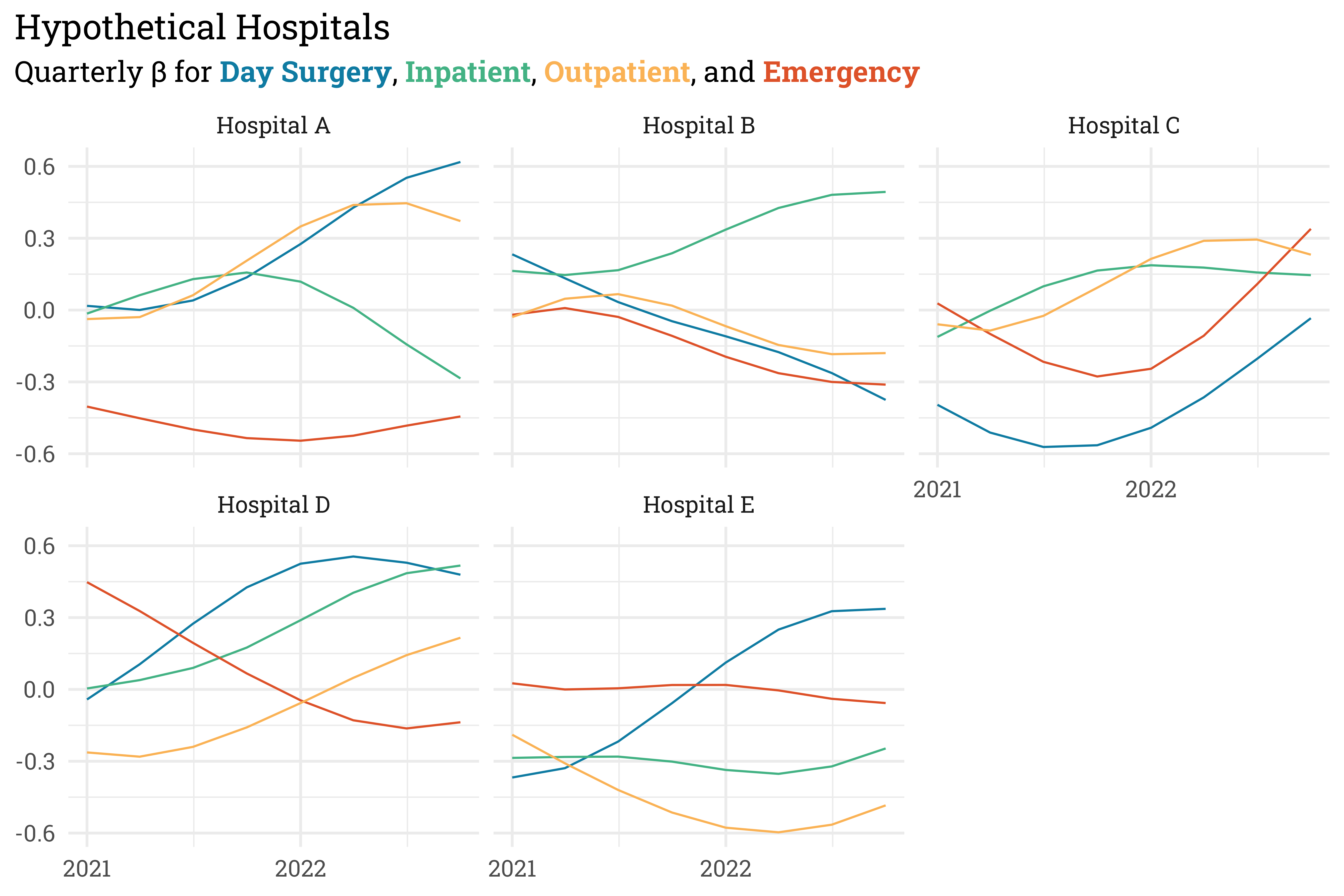These simulated $$\beta_{ij}$$ values will allow for the NPS to slowly vary over time. Let’s flesh out the linear model by adding in the time-invariant terms. Expanding the details below will let you see how the parameters were fixed.

Code
kappa_1 <- -1.5
kappa_2 <- -0.5
sigma_interact <- 0.25
sigma_campus <- 0.15
sigma_service <- 1 # setting to 1 so I can set the z_s parameters manually

set.seed(33)
responses <-
responses %>%

# apply random z-offsets to z_c/z_i
nest(data = -hospital) %>%
bind_cols(z_c = rnorm(nrow(.), 0, 1)) %>%
unnest(data) %>%
nest(data = -c(hospital, service)) %>%
bind_cols(z_i = rnorm(nrow(.), 0, 1)) %>%
unnest(data) %>%

# fix z_s based on domain knowledge
mutate(z_s = case_match(service,
"Inpatient" ~ 0,
"Outpatient" ~ 0.75,
"Day Surgery" ~ 1,
"Emergency" ~ -0.5)) %>%

# apply linear model
mutate(phi = z_c*sigma_campus + z_s*sigma_service + z_i*sigma_interact + beta) %>%
select(hospital:n, phi)

# plot!
responses %>%
ggplot(aes(x = quarter,
y = phi,
color = color)) +
geom_line() +
scale_color_identity() +
scale_x_date(labels = scales::label_date("%Y"),
breaks = "years") +
facet_wrap(~hospital) +
theme_rieke() +
labs(title = "Hypothetical Hospitals",
subtitle = glue::glue("Quarterly \u03D5 for ",
"**{color_text('Day Surgery', col_ds)}**, ",
"**{color_text('Inpatient', col_ip)}**, ",
"**{color_text('Outpatient', col_op)}**, and ",
"**{color_text('Emergency', col_ed)}**"),
x = NULL,
y = NULL)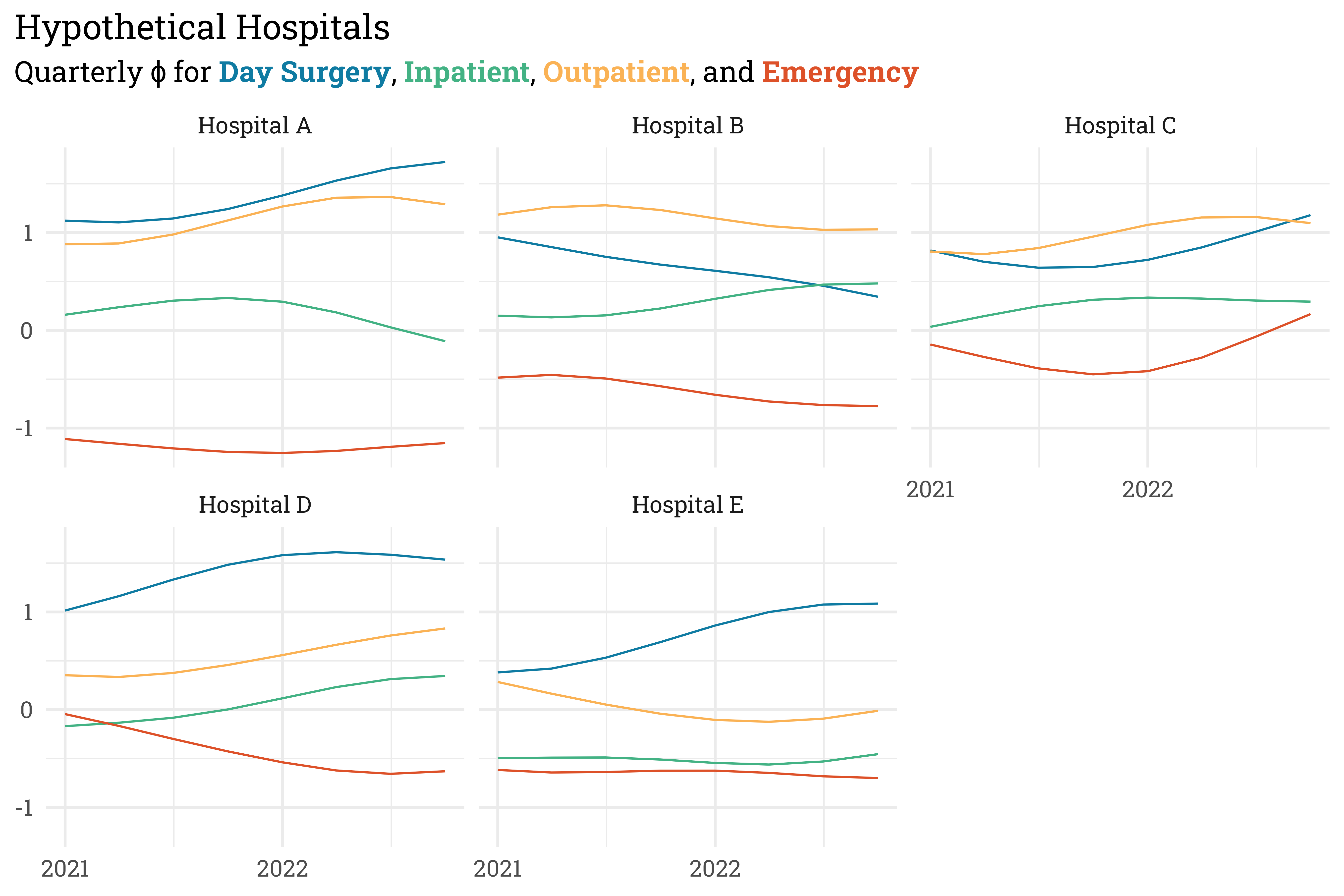Finally, we can push the linear model back through the cumulative probability operations to get the probabilities of each category — promoter, passive, or detractor. NPS is the percentage of promoters minus the percentage of detractors, so we can use these categorical probabilities to get the expected NPS at each quarter. The actual score will deviate (possibly by quite a lot!) from this expected value due to sampling variation.

Code
responses <-
responses %>%
mutate(q1 = kappa_1 - phi,
q2 = kappa_2 - phi,
p1 = expit(q1),
p2 = expit(q2) - expit(q1),
p3 = 1 - expit(q2)) %>%
select(-phi, -q1, -q2)

# plot!
responses %>%
mutate(nps = p3 - p1) %>%
ggplot(aes(x = quarter,
y = nps,
color = color)) +
geom_line() +
facet_wrap(~hospital) +
scale_color_identity() +
scale_x_date(labels = scales::label_date("%Y"),
breaks = "years") +
scale_y_continuous(labels = scales::label_percent(accuracy = 1)) +
theme_rieke() +
labs(title = "Hypothetical Hospitals",
subtitle = glue::glue("Expected NPS for ",
"**{color_text('Day Surgery', col_ds)}**, ",
"**{color_text('Inpatient', col_ip)}**, ",
"**{color_text('Outpatient', col_op)}**, and ",
"**{color_text('Emergency', col_ed)}**"),
x = NULL,
y = NULL)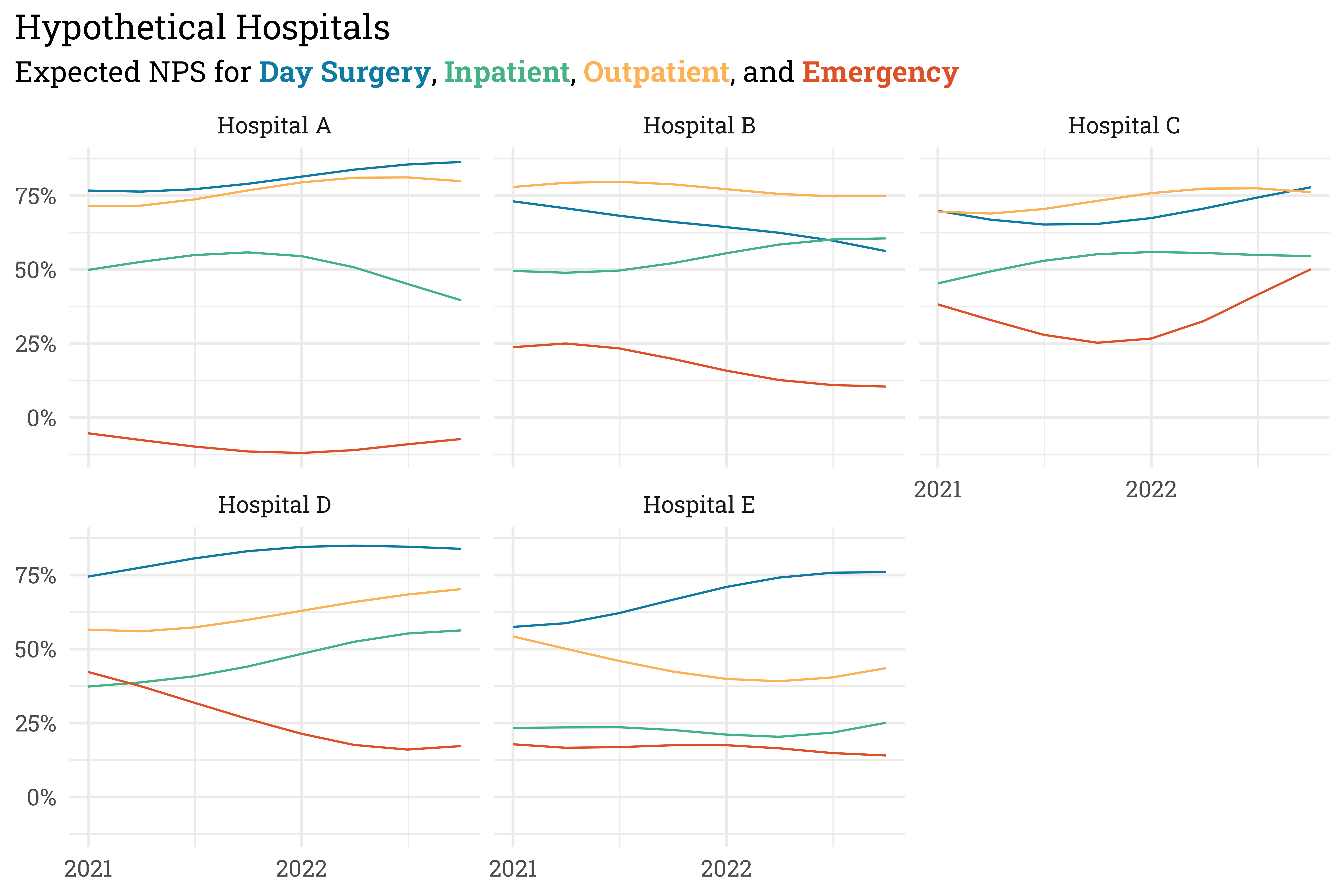Before moving onto the final step, where we simulate actual responses, I want to take a moment just to marvel at the flexibility of gaussian processes — with just a few parameters and a lot math, we can simulate (and on the inverse side, fit a model to) incredibly complex, non-linear data. Let’s see how the responses shake out.

Code
set.seed(34)
responses <-
responses %>%
mutate(responses = pmap(list(n, p1, p2, p3),
~rmultinom(1, ..1, c(..2, ..3, ..4))),
detractor = map_int(responses, ~.x),
passive = map_int(responses, ~.x),
promoter = map_int(responses, ~.x)) %>%
select(-c(p1, p2, p3, responses))

responses %>%
mutate(nps = (promoter - detractor)/n) %>%
ggplot(aes(x = quarter,
y = nps,
color = color)) +
geom_point(aes(size = n),
alpha = 0.75) +
geom_line(alpha = 0.75) +
facet_wrap(~hospital) +
scale_color_identity() +
scale_x_date(labels = scales::label_date("%Y"),
breaks = "years") +
scale_y_continuous(labels = scales::label_percent(accuracy = 1)) +
scale_size_continuous(range = c(1, 4)) +
theme_rieke() +
theme(legend.position = "none") +
labs(title = "Hypothetical Hospitals",
subtitle = glue::glue("Quarterly NPS for ",
"**{color_text('Day Surgery', col_ds)}**, ",
"**{color_text('Inpatient', col_ip)}**, ",
"**{color_text('Outpatient', col_op)}**, and ",
"**{color_text('Emergency', col_ed)}**"),
x = NULL,
y = NULL)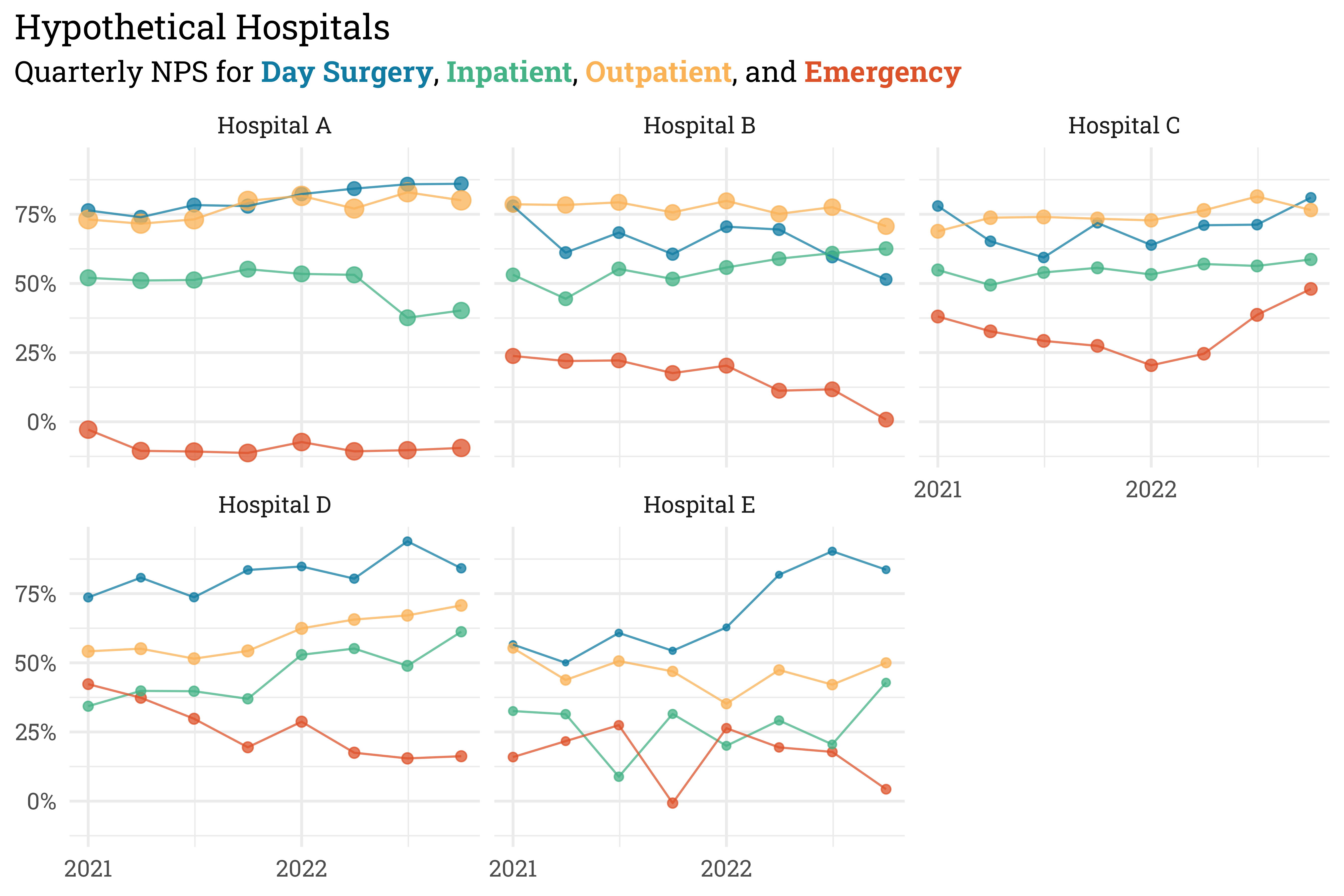With these responses by service area, we can also summarize with a rollup NPS for each hospital (in practice, we create two separate summary scores for services that broadly fall under Inpatient or Outpatient, but in this case we’ll keep it simple and just create one summary score).

Code
responses %>%
group_by(hospital, quarter) %>%
summarise(n = sum(n),
detractor = sum(detractor),
passive = sum(passive),
promoter = sum(promoter)) %>%
ungroup() %>%
mutate(nps = (promoter - detractor)/n) %>%
ggplot(aes(x = quarter,
y = nps)) +
geom_point(aes(size = n),
alpha = 0.75,
color = RColorBrewer::brewer.pal(3, "Dark2")) +
geom_line(alpha = 0.75,
color = RColorBrewer::brewer.pal(3, "Dark2")) +
facet_wrap(~hospital) +
scale_x_date(labels = scales::label_date("%Y"),
breaks = "years") +
scale_y_continuous(labels = scales::label_percent(accuracy = 1)) +
theme_rieke() +
theme(legend.position = "none") +
labs(title = "Hierarchical Hospitals",
subtitle = "Quarterly Rollup NPS at each Hospital",
x = NULL,
y = NULL)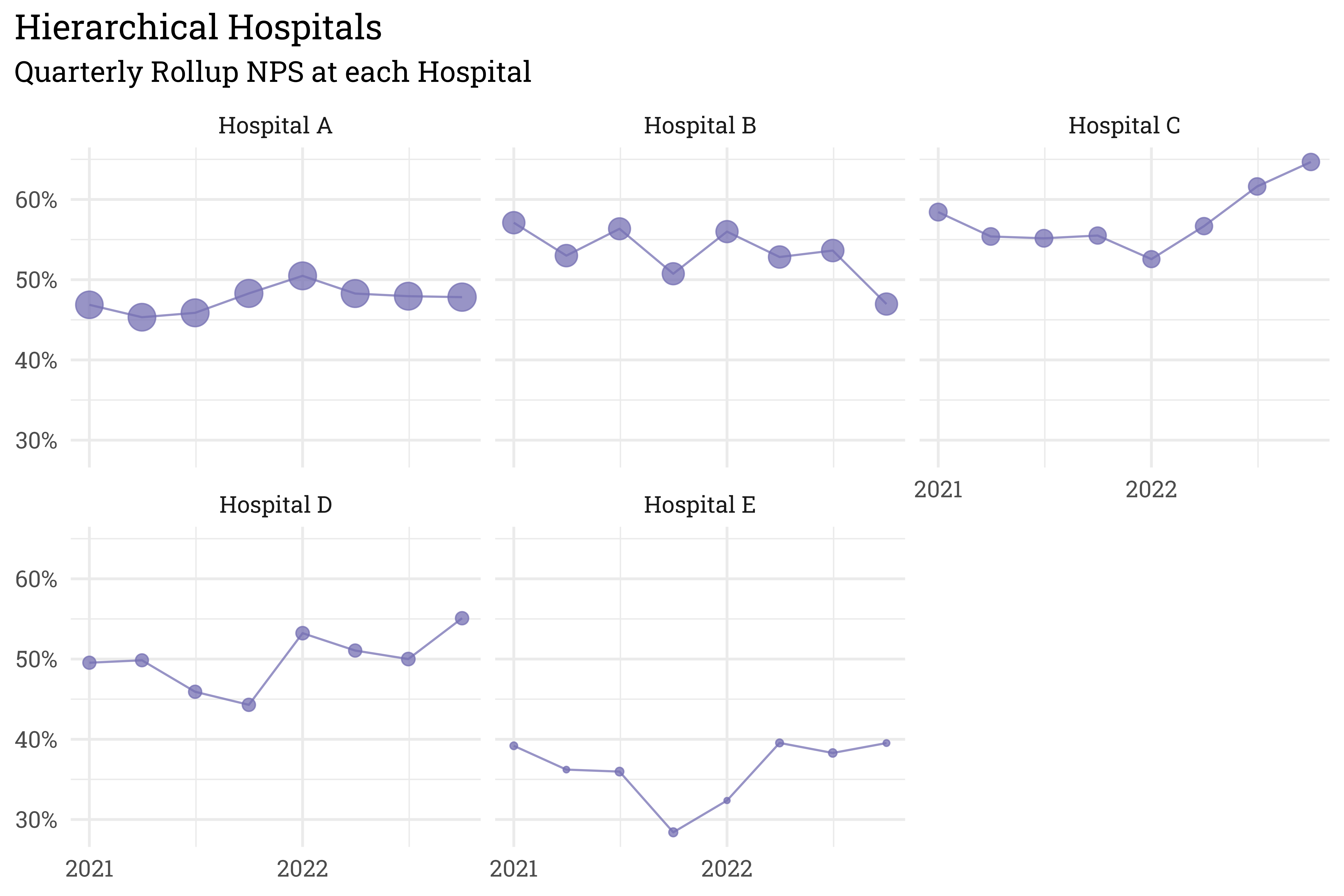And just like that, we have a model for generating NPS at hospital service areas, which we can summarize with an overall NPS for each hospital! Next, I’ll run through the process in reverse by fitting a model to the simulated data. Once I have the estimated parameters, I can look forward and project scores for the upcoming four quarters.

## Like a glove: fitting a model

I’ve written the model in Stan. Stan is great, because it allows you to basically write the mathematical model verbatim in code and the algorithm running underneath the model will automatically warn you when the model is poorly specified/runs into computational issues. You can unfold the code chunks below to see the model verbatim.

Code
# add indicator variables
responses <-
responses %>%

nest(data = -hospital) %>%
bind_cols(cid = seq(1:nrow(.))) %>%
unnest(data) %>%

nest(data = -service) %>%
bind_cols(sid = seq(1:nrow(.))) %>%
unnest(data) %>%

nest(data = -c(hospital, service)) %>%
bind_cols(iid = seq(1:nrow(.))) %>%
unnest(data) %>%

nest(data = -quarter) %>%
bind_cols(qid = seq(1:nrow(.))) %>%
unnest(data)

# create response matrix
R <-
responses %>%
select(detractor, passive, promoter) %>%
as.matrix()

# prep stan data
responses_stan <-
list(
N = nrow(responses),
R = R,
N_services = max(responses$sid), N_campuses = max(responses$cid),
N_interact = max(responses$iid), sid = responses$sid,
cid = responses$cid, iid = responses$iid,
Q = max(responses$qid), distances = seq(from = 0, to = 1, length.out = 12)[1:8], qid = responses$qid
)
Code
data{
int N; // rows in the training set
int R[N, 3]; // number of detractors/passives/promoters at each unit
int N_services; // number of distinct service areas
int N_campuses; // number of distinct campuses
int N_interact; // number of interactions
int sid[N]; // index for service area
int cid[N]; // index for campus
int iid[N]; // index for the interaction term
int Q; // number of quarters considered
real distances[Q]; // vector of time expressed as a distance between 0/1
int qid[N]; // index for quarter
}
parameters{
// probability model
ordered kappa; // cutpoints
real alpha; // global mean
real<lower = 0> sigma_s; // std dev around service
real<lower = 0> sigma_c; // std dev around campus
real<lower = 0> sigma_i; // std dev around interactions
vector[N_services] z_s; // service offset
vector[N_campuses] z_c; // campus offset
vector[N_interact] z_i; // interaction offset

// gaussian process terms
real<lower = 0> amplitude; // 'vertical' scale of gp
real<lower = 0> length_scale; // 'horizontal' scale of gp
matrix[Q, N_interact] eta; // each interaction term gets a column of eta offsets
}
model{
vector p; // probability vector
vector q; // cumulative probability vector
real phi; // linear model term

// additional model terms from gp
matrix[Q, Q] D; // covariance matrix based on distances
matrix[Q, Q] L; // cholesky decomposition of covariance matrix
matrix[Q, N_interact] beta; // quarterly offset for each interaction term

// probability model priors
kappa ~ normal(-1, 0.25);
alpha ~ normal(0, 1);
z_s ~ normal(0, 1);
z_c ~ normal(0, 1);
z_i ~ normal(0, 1);
sigma_s ~ gamma(2, 3);
sigma_c ~ gamma(2, 3);
sigma_i ~ normal(0, 0.5);

// gaussian process priors
amplitude ~ beta(2, 25); // normal 0, 0.1
length_scale ~ beta(2, 10); // normal 0, 0.05
to_vector(eta) ~ normal(0, 1);

// construct distance matrix & cholesky decomposition
for (i in 1:size(distances)) {
D[i,i] = D[i,i] + 1e-9; // not using delta
}
L = cholesky_decompose(D);

// non-centered time-dependent parameter
for (i in 1:N_interact) {
beta[,i] = L * eta[,i];
}

for (i in 1:N) {
// linear model (ignoring time terms)
phi = alpha + z_s[sid[i]]*sigma_s + z_c[cid[i]]*sigma_c + z_i[iid[i]]*sigma_i;

// add in time terms and convert to probability matrix
phi = phi + beta[qid[i], iid[i]];
q = kappa - phi;
q = kappa - phi;
q = inv_logit(q);
p = q;
p = q - q;
p = 1 - q;

// likelihood
R[i,] ~ multinomial(p);
}
}

Let’s fit the model & perform some quick diagnostic checks to make sure everything is sampling as expected.

Code
# this isn't evaluated within this quarto doc
# you can see the output in the repository for this post at the source
response_fit <-
rstan::stan(
"response_model.stan",
data = responses_stan,
chains = 4,
cores = 4,
iter = 2000,
refresh = 100,
seed = 2024,
init_r = 0.1
)
Code
params <- rethinking::precis(response_fit, depth = 3)
index <- order(params[,"Rhat4"], decreasing = TRUE)
params[index[1:10],5:6]
#>                  n_eff    Rhat4
#> sigma_i       902.3297 1.003957
#> length_scale  604.6112 1.003180
#> z_s       1890.4067 1.002883
#> alpha        1709.7763 1.002878
#> z_i       3569.8292 1.002078
#> amplitude     904.6963 1.002040
#> sigma_s      2200.2597 1.001950
#> eta[4,8]     1467.2686 1.001693
#> z_i       2879.9423 1.001668
#> z_s       1812.9102 1.001375

As measured by the convergence statistic, Rhat4, these are the ten parameters that have the worst convergence. All fall well below our rule-of-thumb threshold for concern, 1.01, so we have some pretty good evidence that the model fitting went well. Given that, we can start generating some posterior predictions. The code chunk below walks through the process of generating predictions. It’s a bit lengthy, but essentially just feeds each simulated parameter through the data generating process. There’s a function in there, predict_beta(), that forecasts out into the future by conditioning a multivariate normal on the historical parameters.

Code
posterior_fit <-
response_fit %>%
posterior::as_draws_df() %>%
as_tibble()

# function for extracting id cols as a long format tibble
extract_draws <- function(parameter) {

out <-
posterior_fit %>%
select(.draw, starts_with(parameter)) %>%
pivot_longer(starts_with(parameter),
names_to = paste0(str_sub(parameter, 3), "id"),
values_to = parameter)

out[] <-
as.integer(str_remove_all(out[], paste0(parameter, "\$|\$")))

return(out)

}

sid_draws <- extract_draws("z_s")
cid_draws <- extract_draws("z_c")
iid_draws <- extract_draws("z_i")

# get etas as a nested matrix alongside each draw
eta_draws <-
posterior_fit %>%
select(.draw, starts_with("eta")) %>%
pivot_longer(starts_with("eta"),
names_to = "index",
values_to = "eta") %>%
separate(index, c("row_idx", "col_idx"), ",") %>%
mutate(across(ends_with("idx"), ~as.integer(str_remove_all(.x, "eta\$|\$")))) %>%
pivot_wider(names_from = col_idx,
values_from = eta) %>%
select(-row_idx) %>%
nest(eta = -.draw) %>%
mutate(eta = map(eta, as.matrix))

# filter down to only the needed remaining parameters per draw
posterior_fit <-
posterior_fit %>%
select(alpha,
starts_with("kappa["),
starts_with("sigma"),
amplitude,
length_scale,
.draw)

# function for generating a cholesky factor matrix based on a distance vector
cholesky_distance <- function(x,
amplitude = 1,
length_scale = 1,
delta = 1e-9) {

d <- cov_exp_quad(x, amplitude, length_scale, delta)
L <- chol(d)
return(L)

}

# function for generating a matrix of betas (past, present, and future!)
# from an eta & cholesky matrix
predict_beta <- function(eta, L) {

# find the known betas to condition future betas on
eta_max <- nrow(eta)
L_sub <- L[1:eta_max, 1:eta_max]
x2 <- matrix(0, nrow = nrow(eta), ncol = ncol(eta))

for (i in 1:ncol(eta)) {
x2[,i] <- t(L_sub) %*% eta[,i]
}

# get to MVNormal Sigma from cholesky factor
S <- t(L) %*% L

# separate out S matrix into partitioned matrix
S22 <- S[1:eta_max, 1:eta_max]
S11 <- S[(eta_max + 1):12, (eta_max + 1):12]
S21 <- S[1:eta_max, (eta_max + 1):12]
S12 <- t(S21)

# get MVN distributions for x1 conditional on x2
mu_bar <- S12 %*% MASS::ginv(S22) %*% x2
sigma_bar <- S11 - S12 %*% MASS::ginv(S22) %*% S21

# make predictions for x1 for all cols in eta
x1 <- matrix(0, nrow = 12 - nrow(eta), ncol = ncol(eta))
for (i in 1:ncol(eta)) {
x1[,i] <- MASS::mvrnorm(1, mu_bar[,i], sigma_bar)
}

# combine x1 & x2 into a beta matrix
beta <- matrix(0, nrow = nrow(x2) + nrow(x1), ncol = ncol(x1))
for (i in 1:nrow(x2)) {
beta[i,] <- x2[i,]
}
for (i in 1:nrow(x1)) {
beta[i + nrow(x2),] <- x1[i,]
}

return(beta)

}

# join betas to posterior fit frame
set.seed(35)
posterior_fit <-
posterior_fit %>%
mutate(L = pmap(list(amplitude, length_scale),
~cholesky_distance(seq(from = 0, to = 1, length.out = 12),
..1, ..2))) %>%
left_join(eta_draws) %>%
mutate(beta = pmap(list(eta, L), ~predict_beta(..1, ..2))) %>%
select(-c(amplitude,
length_scale,
L,
eta))

# get one big-ole frame for posterior prediction
posterior_predictions <-
responses %>%
select(-c(quarter,
detractor,
passive,
promoter,
n,
qid)) %>%
distinct() %>%
full_join(iid_draws, by = "iid", multiple = "all") %>%
left_join(cid_draws, by = c("cid", ".draw")) %>%
left_join(sid_draws, by = c("sid", ".draw")) %>%
left_join(posterior_fit, by = ".draw")

# generate predictions
posterior_predictions <-
posterior_predictions %>%

# apply time-invariant linear model
mutate(phi = alpha + z_s*sigma_s + z_c*sigma_c + z_i*sigma_i,
beta = pmap(list(beta, iid), ~..1[,..2]),
quarter = list(seq.Date(from = lubridate::mdy("1/1/21"),
to = lubridate::mdy("10/1/23"),
by = "quarter"))) %>%
unnest(c(quarter, beta)) %>%

# apply time-variance and get probability of each category
mutate(phi = phi + beta,
q1 = kappa - phi,
q2 = kappa - phi,
p1 = expit(q1),
p2 = expit(q2) - expit(q1),
p3 = 1 - expit(q2)) %>%

# join actual response data
select(service,
hospital,
.draw,
quarter,
p1,
p2,
p3) %>%
left_join(responses %>% distinct(service, hospital, quarter, detractor, passive, promoter, n),
by = c("service", "hospital", "quarter"))

# estimate n-size sans model
# this is one of those things I want to include in the next model update
set.seed(36)
posterior_predictions <-
posterior_predictions %>%
mutate(log_n = log(n)) %>%
group_by(service, hospital) %>%
mutate(lambda_mean = mean(log_n, na.rm = TRUE),
lambda_sd = sd(log_n, na.rm = TRUE)) %>%
ungroup() %>%
bind_cols(lambda_sim = rnorm(nrow(.), .$lambda_mean, .$lambda_sd)) %>%
bind_cols(n_sim = rpois(nrow(.), exp(.$lambda_mean))) %>% mutate(n_sim = if_else(is.na(n), n_sim, n)) %>% select(-c(log_n:lambda_sim)) # simulate responses set.seed(37) posterior_predictions <- posterior_predictions %>% mutate(score_sim = pmap(list(n_sim, p1, p2, p3), ~rmultinom(1, ..1, c(..2, ..3, ..4))), nps_sim = map_dbl(score_sim, ~(.x - .x)/sum(.x))) %>% select(service, hospital, .draw, quarter, detractor, passive, promoter, n_sim, nps_sim) %>% nest(sims = c(.draw, n_sim, nps_sim)) posterior_predictions <- posterior_predictions %>% mutate(color = case_match(service, "Inpatient" ~ col_ip, "Outpatient" ~ col_op, "Emergency" ~ col_ed, "Day Surgery" ~ col_ds)) We now have a distribution of possible scores for each service area at each hospital for each quarter. From this distribution, we can set the three goal levels (threshold, target, and distinguished) based on quantiles of the distribution. For conveniences sake, I’ve set these quantiles to 10%, 50%, and 90% for threshold, target, and distinguished, respectively. This means that, according to this model, an area falling below threshold or rising above distinguished is somewhat unlikely to be a fluke and it conveniently lines up with an 80% credible interval. Plotted below, we can see how the scores are expected to shift over time. Code # plot! posterior_predictions %>% mutate(.pred_nps = map_dbl(sims, ~quantile(.x$nps_sim, probs = 0.5)),
.pred_nps_lower = map_dbl(sims, ~quantile(.x$nps_sim, probs = 0.1)), .pred_nps_upper = map_dbl(sims, ~quantile(.x$nps_sim, probs = 0.9)),
n = promoter + passive + detractor,
nps = (promoter - detractor)/n) %>%
ggplot(aes(x = quarter,
y = .pred_nps,
ymin = .pred_nps_lower,
ymax = .pred_nps_upper)) +
geom_vline(xintercept = lubridate::mdy("1/1/23"),
linetype = "dashed",
color = "gray60",
alpha = 0.5) +
geom_ribbon(aes(fill = color),
alpha = 0.5) +
geom_line(aes(color = color),
linewidth = 0.25) +
scale_fill_identity() +
scale_color_identity() +
scale_y_continuous(labels = scales::label_percent(accuracy = 1)) +
facet_wrap(~hospital) +
theme_rieke() +
labs(title = "Hypothetical Hospitals",
subtitle = glue::glue("Predicted NPS for ",
"**{color_text('Day Surgery', col_ds)}**, ",
"**{color_text('Inpatient', col_ip)}**, ",
"**{color_text('Outpatient', col_op)}**, and ",
"**{color_text('Emergency', col_ed)}**"),
x = NULL,
y = NULL,
caption = paste("Posterior prediction from 4,000 MCMC samples",
"Shaded range represents 80% credible interval",
sep = "<br>"))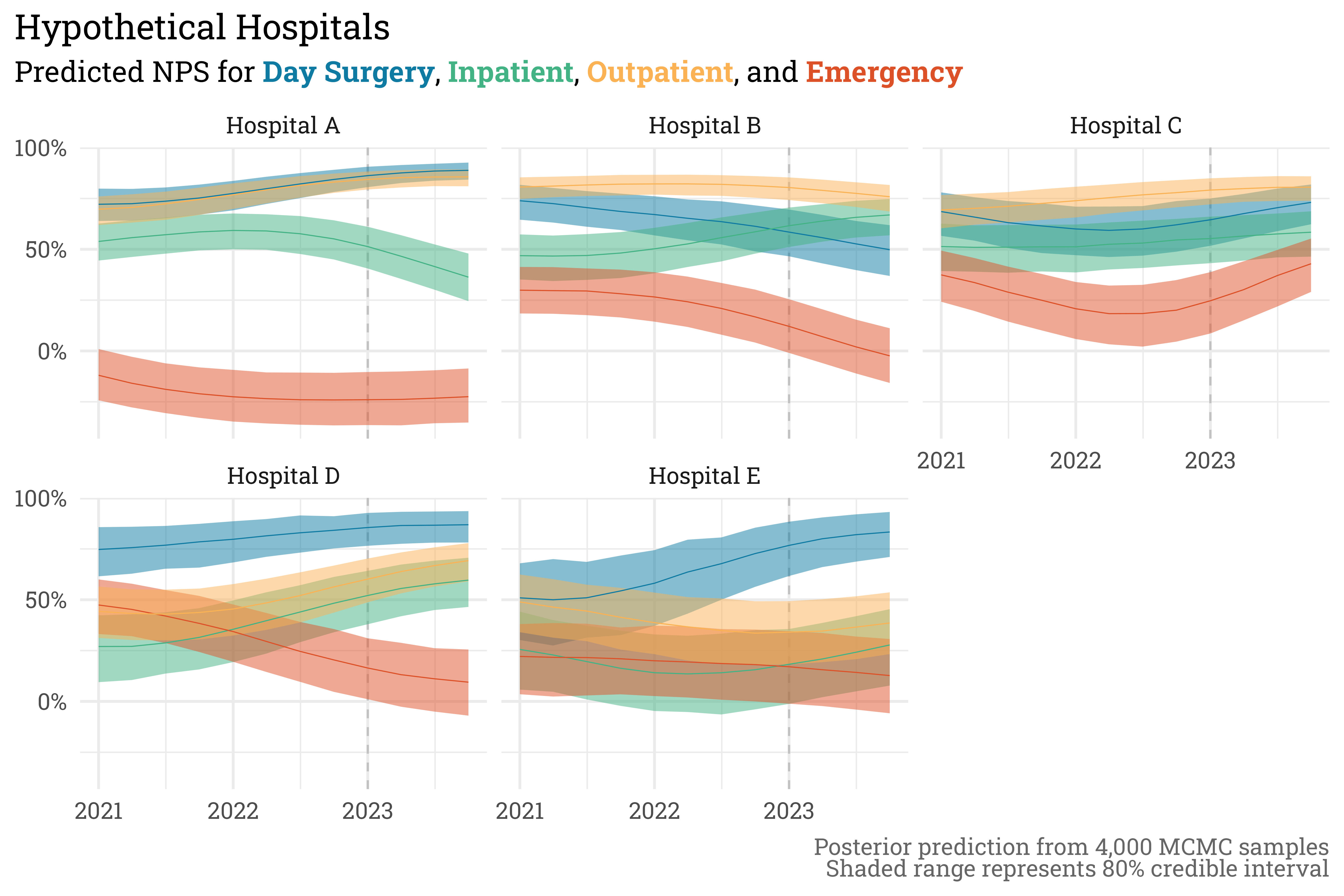We can also summarize the predictions in rollup scores at each hospital.

Code
posterior_predictions %>%
unnest(sims) %>%
select(hospital, quarter, .draw, n_sim, nps_sim) %>%
group_by(hospital, quarter, .draw) %>%
summarise(nps = sum((nps_sim * n_sim))/sum(n_sim)) %>%
group_by(hospital, quarter) %>%
summarise(.pred_nps = quantile(nps, probs = 0.5),
.pred_nps_lower = quantile(nps, probs = 0.1),
.pred_nps_upper = quantile(nps, probs = 0.9)) %>%
ungroup() %>%
ggplot(aes(x = quarter,
y = .pred_nps,
ymin = .pred_nps_lower,
ymax = .pred_nps_upper)) +
geom_vline(xintercept = lubridate::mdy("1/1/23"),
linetype = "dashed",
color = "gray60",
alpha = 0.5) +
geom_ribbon(fill = RColorBrewer::brewer.pal(3, "Dark2"),
alpha = 0.5) +
geom_line(color = RColorBrewer::brewer.pal(3, "Dark2"),
linewidth = 0.25) +
facet_wrap(~hospital) +
scale_y_continuous(labels = scales::label_percent(accuracy = 1)) +
theme_rieke() +
labs(title = "Hypothetical Hospitals",
subtitle = "Predicted Rollup NPS at each Hospital",
x = NULL,
y = NULL,
caption = paste("Posterior prediction from 4,000 MCMC samples",
"Shaded range represents 80% credible interval",
sep = "<br>"))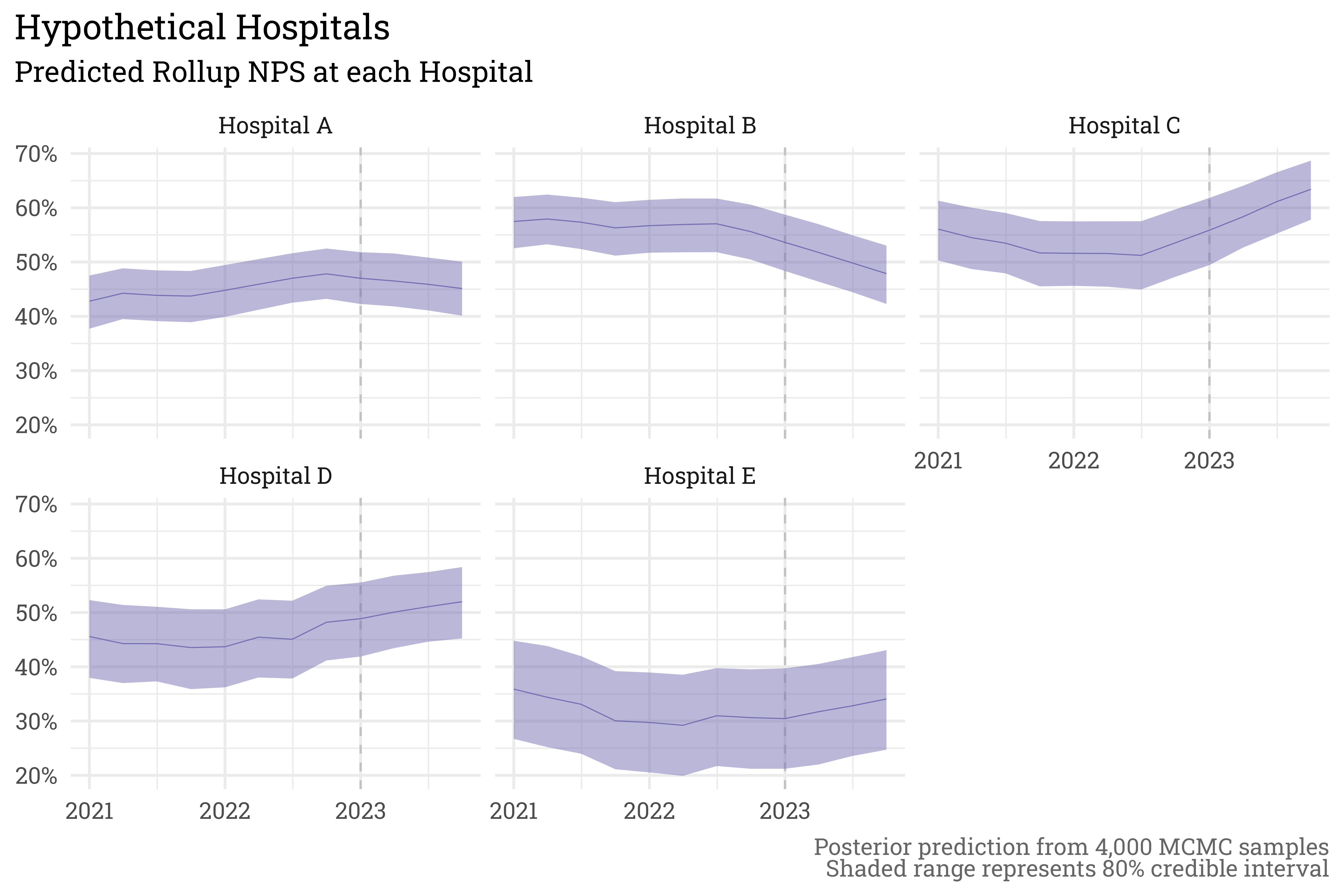The $$\sigma_{length}^2$$ parameter used to simulate data implies that scores take quite a bit of time to return to their stabilized value — here it appears four quarters in 2023 isn’t lengthy enough to reach stability. Despite this, the gaussian process allows us to predict how scores will progress through the future as they decay towards their stabilized value. In our actual goal predictions file, we also summarize the scores at an annual level and present the results in a table. It’s a bit late on a Sunday afternoon and I’ve been staring at code for the better part of a day, so I’ll instead I’ll just leave it at the plot.

## Some closing thoughts

Andrew Gelman has a fun phrase that he uses often — “big data means big model.” With years of patient satisfaction data to sift through, we certainly have “big data,” and gaussian processes provide the means for fitting a big, complex model to the data. This complexity comes at a cost. Taming the real goal model took a lot of time and effort, and there’s still more work to do (which probably means I’ll end up needing to refactor the model again). That being said, the juice here is worth the squeeze — gaussian processes are a powerful, flexible tool that allow us to express complex ideas with just a lil bit of matrix math.

## Citation

BibTeX citation:
@online{rieke2023,
author = {Rieke, Mark},
title = {Gauss’ {Goal} {Generation}},
date = {2023-03-19},